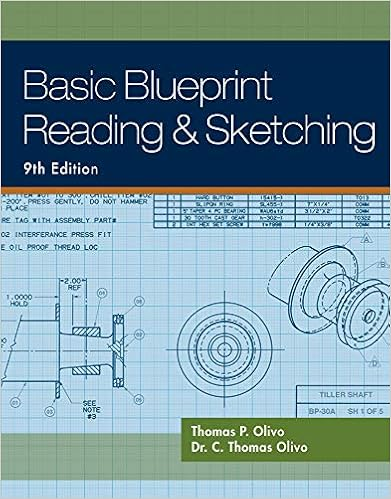# D transform faults there are several transform faults

• Notes
• mignonaaka0011
• 15
• 51% (37) 19 out of 37 people found this document helpful

This preview shows page 4 - 7 out of 15 pages.

##### We have textbook solutions for you!
The document you are viewing contains questions related to this textbook.The document you are viewing contains questions related to this textbook.
Chapter 4 / Exercise 12
OlivoExpert Verified
4 “Geoworld” Plate Tectonics Lab D. Transform Faults There are several transform faults in the Elrond Sea. The only parts of these faults that are presently active are the parts between the segments of the Hobbit Ridge. 1. Using arrows, show the sense of motion on each of the active transform faults on the map. 2. In the space below, draw a diagram and explain why the sense of motion on a transform fault is the way it is. E. Earthquakes 1. Describe three places on the planet of Geoworld where you would expect large numbers of earthquakes. a. b. c. 2. The vast majority of earthquakes are caused by movement on faults. What types of faults would you expect at each location you described above (thrust, normal, strike- slip)? a. b. c. 3. For each location described above, record whether the earthquakes will have deep foci and/or shallow foci. a. b. c.
##### We have textbook solutions for you!
The document you are viewing contains questions related to this textbook.The document you are viewing contains questions related to this textbook.
Chapter 4 / Exercise 12Expert Verified
•••